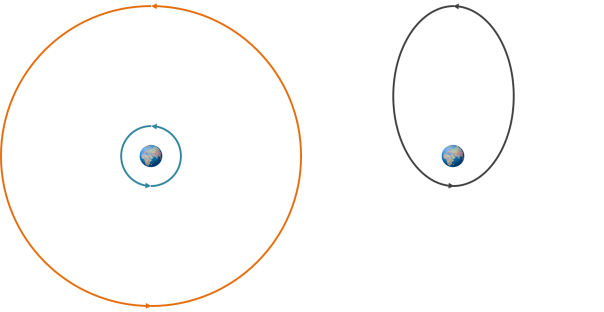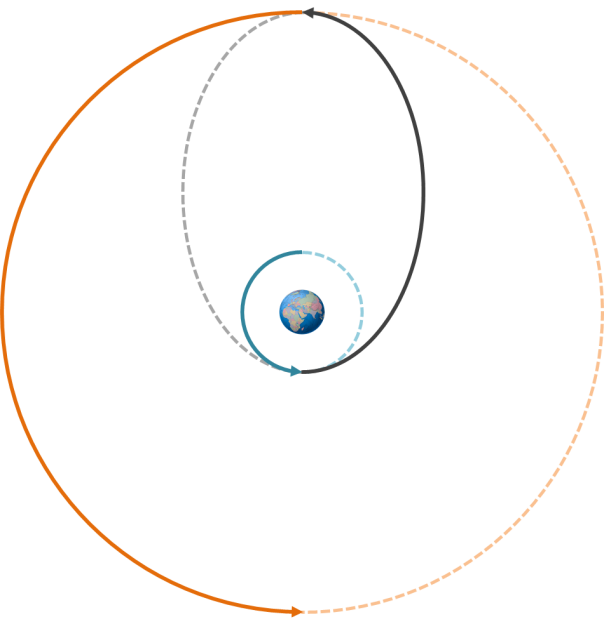# Hohmann Transfers

The Hohmann transfer is an orbital manoeuvre used to transfer a satellite between two different circular orbits.On the left, the two circular orbits between which the transfer will take place. On the right, the elliptical Hohmann transfer orbit.

The orbit of the lower (blue) orbit has the lowest energy (i.e. the specific orbital energy) of the three, the Hohmann transfer orbit has a higher energy than that, and the highest (orange) orbit has the greatest energy of the three.  The gravitational potential and kinetic energies of the initial and final circular orbits are fairly constant, but the gravitational potential and kinetic energies of the Hohmann transfer orbit vary substantially as the orbiting object transfers gravitational potential to kinetic as it approaches Earth and vice versa.The Hohmann transfer takes place along (half of) an elliptical orbit with one half of the ellipse touching the lower orbit and the other half touching the higher orbit. Two different thruster impulses are used: one to move it onto the elliptical orbit, and then a second one to move it onto the higher orbit. Each time the thruster is fired this increases the kinetic energy of the satellite, which is then transferred to the gravitational potential energy of its new orbit. Because orbits are reversible, moving from a higher orbit to a lower orbit still involves two impulses, but they are in a direction opposite to the motion of the satellite, causing it to decrease in speed and “fall” into the lower orbit.